title: ‘Data 606 Chapter 6: Inference for categorical data’

author: ‘Sufian’

output:

html_document:

css: ./lab.css

highlight: pygments

theme: cerulean

pdf_document: default

http://rpubs.com/ssufian/541162

In August of 2012, news outlets ranging from the Washington Post to the Huffington Post ran a story about the rise of atheism in America. The source for the story was a poll that asked people, “Irrespective of whether you attend a place of worship or not, would you say you are a religious person, not a religious person or a convinced atheist?” This type of question, which asks people to classify themselves in one way or another, is common in polling and generates categorical data. In this lab we take a look at the atheism survey and explore what’s at play when making inference about population proportions using categorical data.

## The survey

To access the press release for the poll, conducted by WIN-Gallup International, click on the following link:

https://github.com/jbryer/DATA606/blob/master/inst/labs/Lab6/more/Global_INDEX_of_Religiosity_and_Atheism_PR__6.pdf

Take a moment to review the report then address the following questions.

1. In the first paragraph, several key findings are reported. Do these percentages appear to be sample statistics (derived from the data sample) or population parameters?

ans:

These findings are sample statistics derived from the surveys used to estimate population proportions

2. The title of the report is “Global Index of Religiosity and Atheism”. To generalize the report’s findings to the global human population, what must we assume about the sampling method? Does that seem like a reasonable assumption?

ans:

We must assume that the findings were:

1. random sample from the entire population,

2. the groups are independent,

3. the sample size should be sufficiently large enough.

## The data

Turn your attention to Table 6 (pages 15 and 16), which reports the sample size and response percentages for all 57 countries. While this is a useful format to summarize the data, we will base our analysis on the original data set of individual responses to the survey. Load this data set into R with the following command.

#load("more/atheism.RData")

load(url("http://www.openintro.org/stat/data/atheism.RData"))
1. What does each row of Table 6 correspond to? What does each row of atheism correspond to?

ans:

In table 6, each row correspond to observations at country level and each row of “atheism” correspond to

observations at people level

To investigate the link between these two ways of organizing this data, take a look at the estimated proportion of atheists in the United States. Towards the bottom of Table 6, we see that this is 5%. We should be able to come to the same number using the atheism data.

1. Using the command below, create a new dataframe called us12 that contains only the rows in atheism associated with respondents to the 2012 survey from the United States. Next, calculate the proportion of atheist responses. Does it agree with the percentage in Table 6? If not, why?
library(dplyr)
##
## Attaching package: 'dplyr'
## The following objects are masked from 'package:stats':
##
##     filter, lag
## The following objects are masked from 'package:base':
##
##     intersect, setdiff, setequal, union
us12 <- subset(atheism, nationality == "United States" & year == "2012")
head(atheism)
##   nationality    response year
## 1 Afghanistan non-atheist 2012
## 2 Afghanistan non-atheist 2012
## 3 Afghanistan non-atheist 2012
## 4 Afghanistan non-atheist 2012
## 5 Afghanistan non-atheist 2012
## 6 Afghanistan non-atheist 2012
head(us12 , n=11)
##         nationality    response year
## 49926 United States non-atheist 2012
## 49927 United States non-atheist 2012
## 49928 United States non-atheist 2012
## 49929 United States non-atheist 2012
## 49930 United States non-atheist 2012
## 49931 United States non-atheist 2012
## 49932 United States non-atheist 2012
## 49933 United States non-atheist 2012
## 49934 United States non-atheist 2012
## 49935 United States non-atheist 2012
## 49936 United States non-atheist 2012
us12new <- atheism %>%
filter(nationality == "United States" & year == "2012")

us12new1 <- table(us12new )

prop <-  prop.table(us12new1 )

prop <- data.frame(prop) %>%
filter (nationality == "United States")

prop
##     nationality    response year      Freq
## 1 United States     atheist 2012 0.0499002
## 2 United States non-atheist 2012 0.9500998

ans:

As calculated, Yes, the proportion of atheists in the United States is 0.0499002 which is close to 5%

## Inference on proportions

As was hinted at in Exercise 1, Table 6 provides statistics, that is, calculations made from the sample of 51,927 people. What we’d like, though, is insight into the population parameters. You answer the question, “What proportion of people in your sample reported being atheists?” with a statistic; while the question “What proportion of people on earth would report being atheists” is answered with an estimate of the parameter.

The inferential tools for estimating population proportion are analogous to those used for means in the last chapter: the confidence interval and the hypothesis test.

1. Write out the conditions for inference to construct a 95% confidence interval for the proportion of atheists in the United States in 2012. Are you confident all conditions are met?

ans:

• Observations must be random and independent.

• The assumptions of normality hold for proportions, np >10. Since the US Sample size is 1002, this is easily

met

• The success-failure conditions require np >= 10 and n(1-p) >= 10.

If the conditions for inference are reasonable, we can either calculate the standard error and construct the interval by hand, or allow the inference function to do it for us.

inference(us12$response, est = "proportion", type = "ci", method = "theoretical", success = "atheist") ## Single proportion -- success: atheist ## Summary statistics: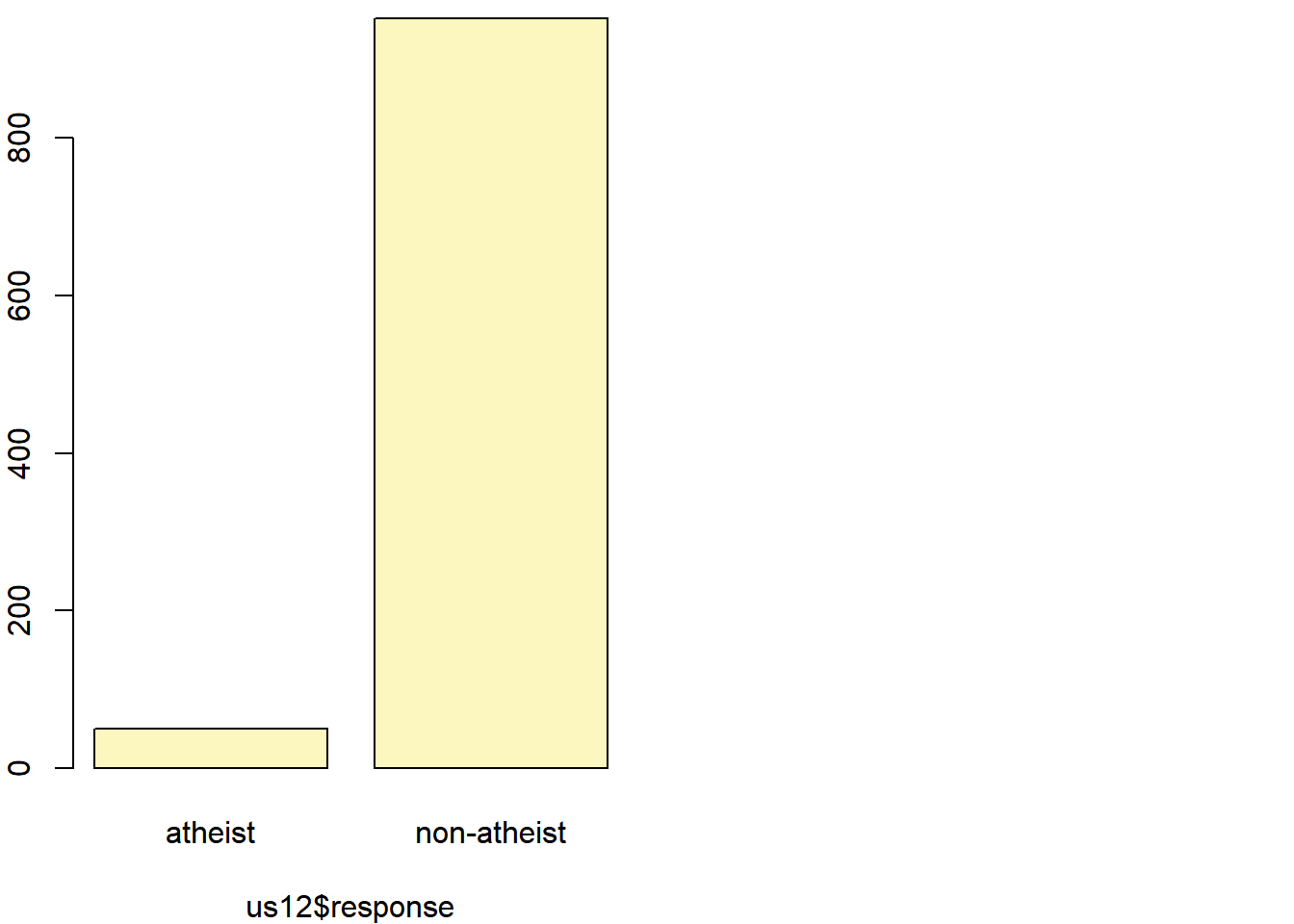## p_hat = 0.0499 ; n = 1002 ## Check conditions: number of successes = 50 ; number of failures = 952 ## Standard error = 0.0069 ## 95 % Confidence interval = ( 0.0364 , 0.0634 ) Note that since the goal is to construct an interval estimate for a proportion, it’s necessary to specify what constitutes a “success”, which here is a response of "atheist". Although formal confidence intervals and hypothesis tests don’t show up in the report, suggestions of inference appear at the bottom of page 7: “In general, the error margin for surveys of this kind is $$\pm$$ 3-5% at 95% confidence”. 1. Based on the R output, what is the margin of error for the estimate of the proportion of the proportion of atheists in US in 2012? ans: Margin of Error = 0.0135, see below z <- 1.96 SE <- 0.0069 ME <- z * SE ME ##  0.013524 1. Using the inference function, calculate confidence intervals for the proportion of atheists in 2012 in two other countries of your choice, and report the associated margins of error. Be sure to note whether the conditions for inference are met. It may be helpful to create new data sets for each of the two countries first, and then use these data sets in the inference function to construct the confidence intervals. #country Argentina arg12 <- subset(atheism, nationality == "Argentina" & year == "2012") head(arg12 , n=11) ## nationality response year ## 1032 Argentina non-atheist 2012 ## 1033 Argentina non-atheist 2012 ## 1034 Argentina non-atheist 2012 ## 1035 Argentina non-atheist 2012 ## 1036 Argentina non-atheist 2012 ## 1037 Argentina non-atheist 2012 ## 1038 Argentina non-atheist 2012 ## 1039 Argentina non-atheist 2012 ## 1040 Argentina non-atheist 2012 ## 1041 Argentina non-atheist 2012 ## 1042 Argentina non-atheist 2012 arg12new <- atheism %>% filter(nationality == "Argentina" & year == "2012") arg12new1<- table(arg12new) proparg <- prop.table(arg12new1) proparg <- data.frame(proparg ) %>% filter (nationality == "Argentina") #country Brazil bra12 <- subset(atheism, nationality == "Brazil" & year == "2012") head(bra12 , n=11) ## nationality response year ## 6595 Brazil non-atheist 2012 ## 6596 Brazil non-atheist 2012 ## 6597 Brazil non-atheist 2012 ## 6598 Brazil non-atheist 2012 ## 6599 Brazil non-atheist 2012 ## 6600 Brazil non-atheist 2012 ## 6601 Brazil non-atheist 2012 ## 6602 Brazil non-atheist 2012 ## 6603 Brazil non-atheist 2012 ## 6604 Brazil non-atheist 2012 ## 6605 Brazil non-atheist 2012 bra12new <- atheism %>% filter(nationality == "Brazil" & year == "2012") bra12new1<- table(bra12new) propbra<- prop.table(bra12new1) propbra <- data.frame(propbra) %>% filter (nationality == "Brazil") proparg ## nationality response year Freq ## 1 Argentina atheist 2012 0.07063572 ## 2 Argentina non-atheist 2012 0.92936428 propbra ## nationality response year Freq ## 1 Brazil atheist 2012 0.00999001 ## 2 Brazil non-atheist 2012 0.99000999 #inferences for Brazil inference(bra12$response, est = "proportion", type = "ci", method = "theoretical",
success = "atheist")
## Single proportion -- success: atheist
## Summary statistics: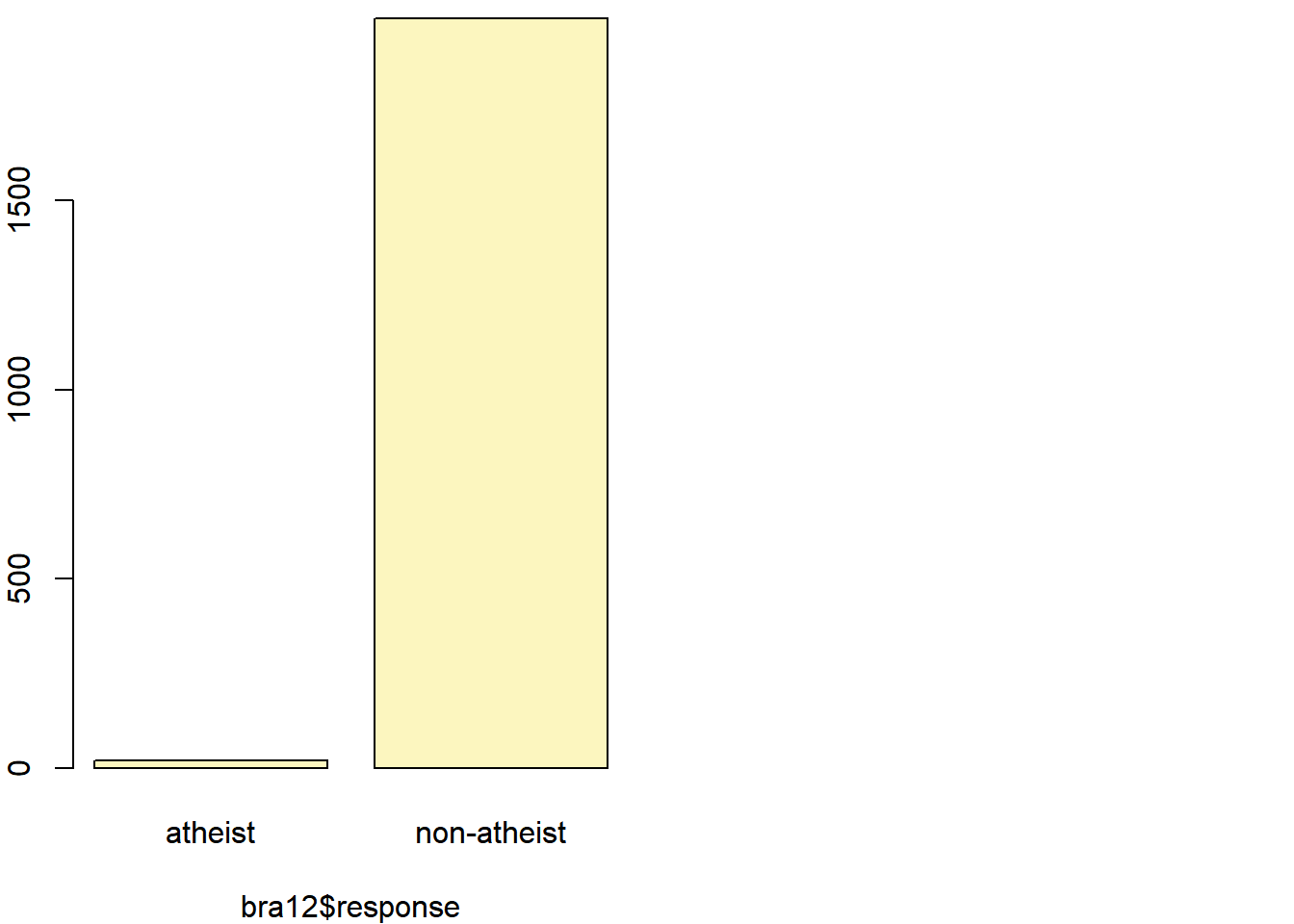## p_hat = 0.01 ;  n = 2002
## Check conditions: number of successes = 20 ; number of failures = 1982
## Standard error = 0.0022
## 95 % Confidence interval = ( 0.0056 , 0.0143 )
z <- 1.96 # using the same confidence level as in previous example
SEbra <- 0.0022
MEbra <- z * SEbra
MEbra
##  0.004312
#inferences for Argentina

inference(arg12 $response, est = "proportion", type = "ci", method = "theoretical", success = "atheist") ## Single proportion -- success: atheist ## Summary statistics: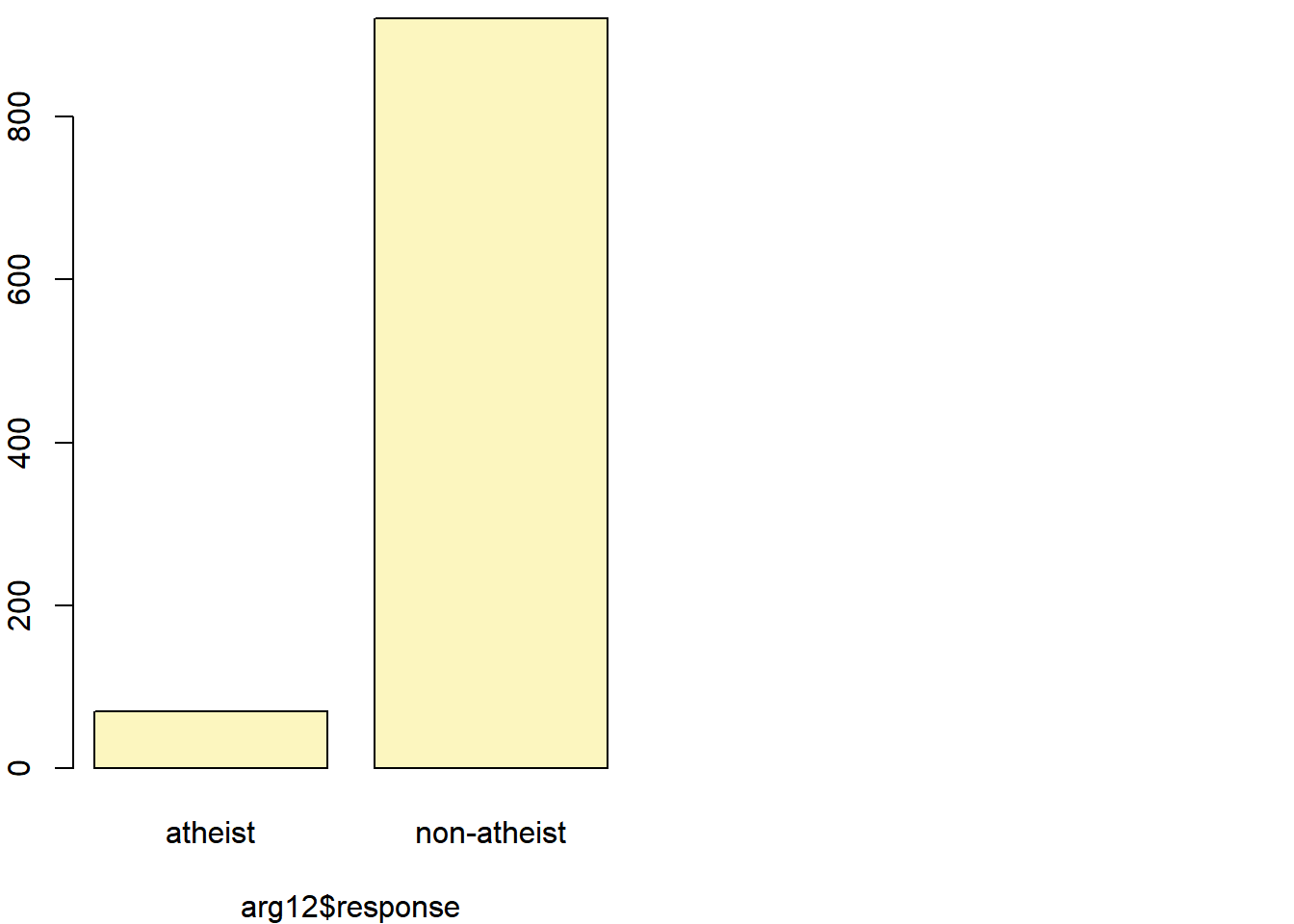## p_hat = 0.0706 ; n = 991 ## Check conditions: number of successes = 70 ; number of failures = 921 ## Standard error = 0.0081 ## 95 % Confidence interval = ( 0.0547 , 0.0866 ) z <- 1.96 # using the same confidence level as in previous example SEarg <- 0.0081 MEarg <- z * SEarg MEarg ##  0.015876 ans: For Brazil, margin of error is 0.004312 For Argentina, margin of error is 0.015876 The conditions for the inference for Brazil and Argentina are met. The sample size are much less than 10% of the total populations for each country. The sample sizes are big enough to satisfy the Success_failure condition. ## How does the proportion affect the margin of error? Imagine you’ve set out to survey 1000 people on two questions: are you female? and are you left-handed? Since both of these sample proportions were calculated from the same sample size, they should have the same margin of error, right? Wrong! While the margin of error does change with sample size, it is also affected by the proportion. Think back to the formula for the standard error: $$SE = \sqrt{p(1-p)/n}$$. This is then used in the formula for the margin of error for a 95% confidence interval: $$ME = 1.96\times SE = 1.96\times\sqrt{p(1-p)/n}$$. Since the population proportion $$p$$ is in this $$ME$$ formula, it should make sense that the margin of error is in some way dependent on the population proportion. We can visualize this relationship by creating a plot of $$ME$$ vs. $$p$$. The first step is to make a vector p that is a sequence from 0 to 1 with each number separated by 0.01. We can then create a vector of the margin of error (me) associated with each of these values of p using the familiar approximate formula ($$ME = 2 \times SE$$). Lastly, we plot the two vectors against each other to reveal their relationship. n <- 1000 p <- seq(0, 1, 0.01) me <- 2 * sqrt(p * (1 - p)/n) plot(me ~ p, ylab = "Margin of Error", xlab = "Population Proportion")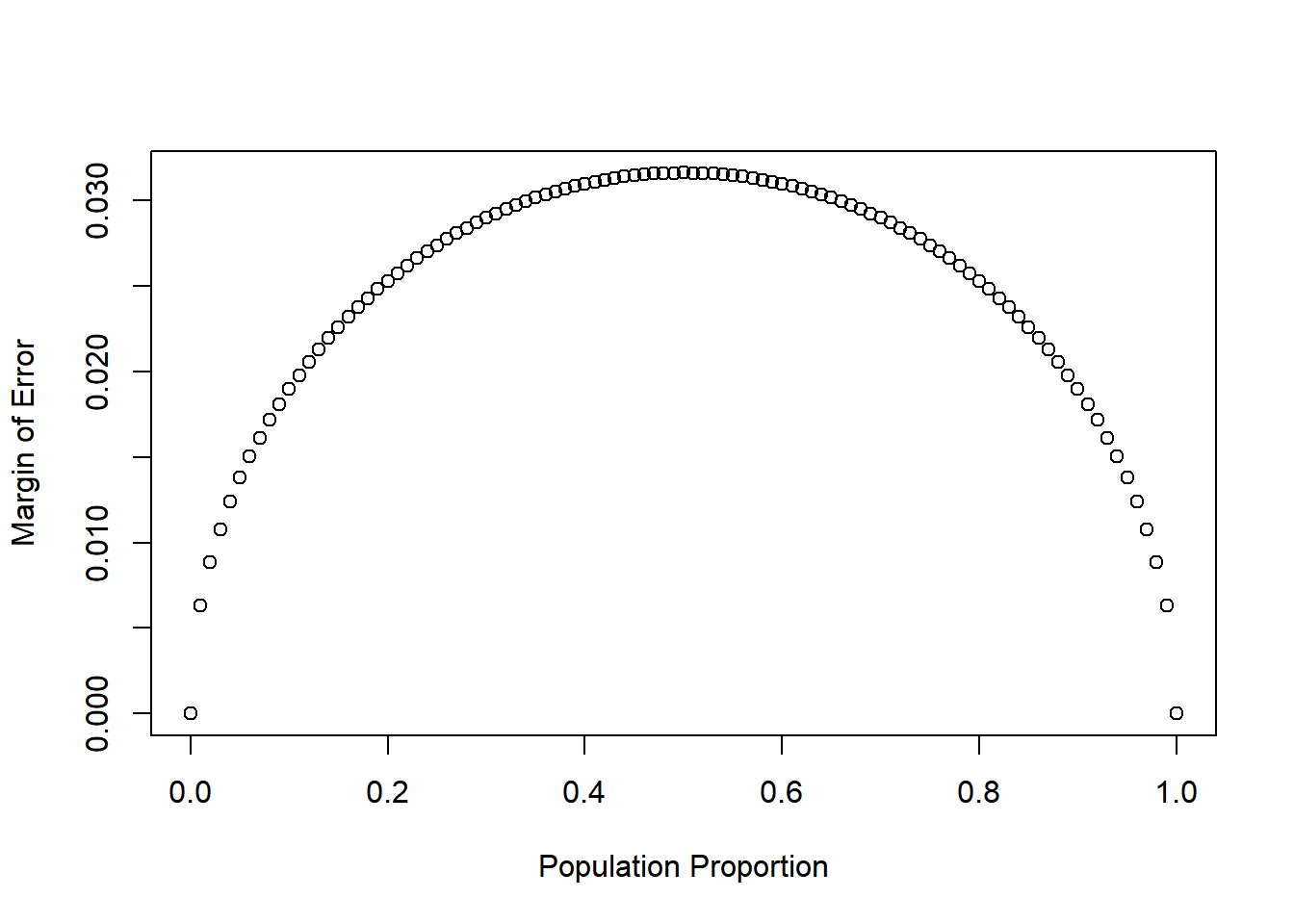1. Describe the relationship between p and me. ans: The margin of error is a function of Population proportion and it reaches its max when proportion is 0.5  However, at both ends, the Margin of error is the smallest. ## Success-failure condition The textbook emphasizes that you must always check conditions before making inference. For inference on proportions, the sample proportion can be assumed to be nearly normal if it is based upon a random sample of independent observations and if both $$np \geq 10$$ and $$n(1 - p) \geq 10$$. This rule of thumb is easy enough to follow, but it makes one wonder: what’s so special about the number 10? The short answer is: nothing. You could argue that we would be fine with 9 or that we really should be using 11. What is the “best” value for such a rule of thumb is, at least to some degree, arbitrary. However, when $$np$$ and $$n(1-p)$$ reaches 10 the sampling distribution is sufficiently normal to use confidence intervals and hypothesis tests that are based on that approximation. We can investigate the interplay between $$n$$ and $$p$$ and the shape of the sampling distribution by using simulations. To start off, we simulate the process of drawing 5000 samples of size 1040 from a population with a true atheist proportion of 0.1. For each of the 5000 samples we compute $$\hat{p}$$ and then plot a histogram to visualize their distribution. p <- 0.1 n <- 1040 p_hats <- rep(0, 5000) for(i in 1:5000){ samp <- sample(c("atheist", "non_atheist"), n, replace = TRUE, prob = c(p, 1-p)) p_hats[i] <- sum(samp == "atheist")/n } hist(p_hats, main = "p = 0.1, n = 1040", xlim = c(0, 0.18))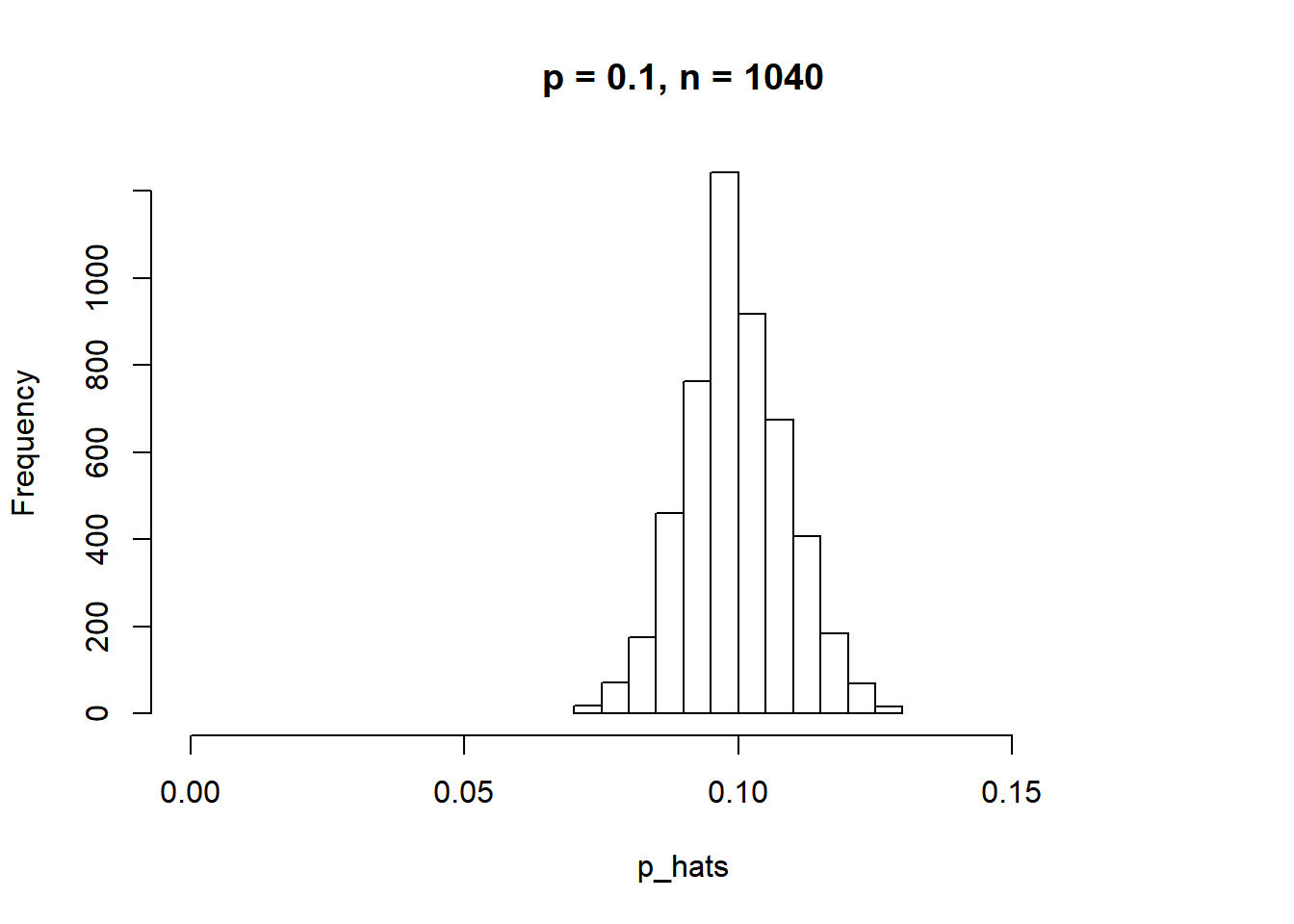These commands build up the sampling distribution of $$\hat{p}$$ using the familiar for loop. You can read the sampling procedure for the first line of code inside the for loop as, “take a sample of size $$n$$ with replacement from the choices of atheist and non-atheist with probabilities $$p$$ and $$1 - p$$, respectively.” The second line in the loop says, “calculate the proportion of atheists in this sample and record this value.” The loop allows us to repeat this process 5,000 times to build a good representation of the sampling distribution. 1. Describe the sampling distribution of sample proportions at $$n = 1040$$ and $$p = 0.1$$. Be sure to note the center, spread, and shape. Hint: Remember that R has functions such as mean to calculate summary statistics. ans: center : 0.09 spread : 0.01 shape : is normal with skew of 0.06 and kurtosis of -0.09 Based on these statistic, the sampling distribution is virtually normal library(psych) describe(p_hats) ## vars n mean sd median trimmed mad min max range skew kurtosis ## X1 1 5000 0.1 0.01 0.1 0.1 0.01 0.07 0.13 0.06 0.06 -0.09 ## se ## X1 0 summary(p_hats) ## Min. 1st Qu. Median Mean 3rd Qu. Max. ## 0.07019 0.09327 0.09904 0.09969 0.10577 0.12981 1. Repeat the above simulation three more times but with modified sample sizes and proportions: for $$n = 400$$ and $$p = 0.1$$, $$n = 1040$$ and $$p = 0.02$$, and $$n = 400$$ and $$p = 0.02$$. Plot all four histograms together by running the par(mfrow = c(2, 2)) command before creating the histograms. You may need to expand the plot window to accommodate the larger two-by-two plot. Describe the three new sampling distributions. Based on these limited plots, how does $$n$$ appear to affect the distribution of $$\hat{p}$$? How does $$p$$ affect the sampling distribution? part a): n=400 & p 0.1 p <- 0.1 n <- 400 p_hats1 <- rep(0, 5000) for(i in 1:5000){ samp <- sample(c("atheist", "non_atheist"), n, replace = TRUE, prob = c(p, 1-p)) p_hats1[i] <- sum(samp == "atheist")/n } part b): n=1040 & p 0.02 p <- 0.02 n <- 1040 p_hats2 <- rep(0, 5000) for(i in 1:5000){ samp <- sample(c("atheist", "non_atheist"), n, replace = TRUE, prob = c(p, 1-p)) p_hats2[i] <- sum(samp == "atheist")/n } part c): n=400 & p 0.02 p <- 0.02 n <- 400 p_hats3 <- rep(0, 5000) for(i in 1:5000){ samp <- sample(c("atheist", "non_atheist"), n, replace = TRUE, prob = c(p, 1-p)) p_hats3[i] <- sum(samp == "atheist")/n } par(mfrow = c(2, 2)) hist(p_hats, main = "p = 0.1, n = 1040", xlim = c(0, 0.18)) hist(p_hats1, main = "p = 0.1, n = 400", xlim = c(0, 0.18)) hist(p_hats2, main = "p = 0.02, n = 1040", xlim = c(0, 0.18)) hist(p_hats3, main = "p = 0.02, n = 400", xlim = c(0, 0.18))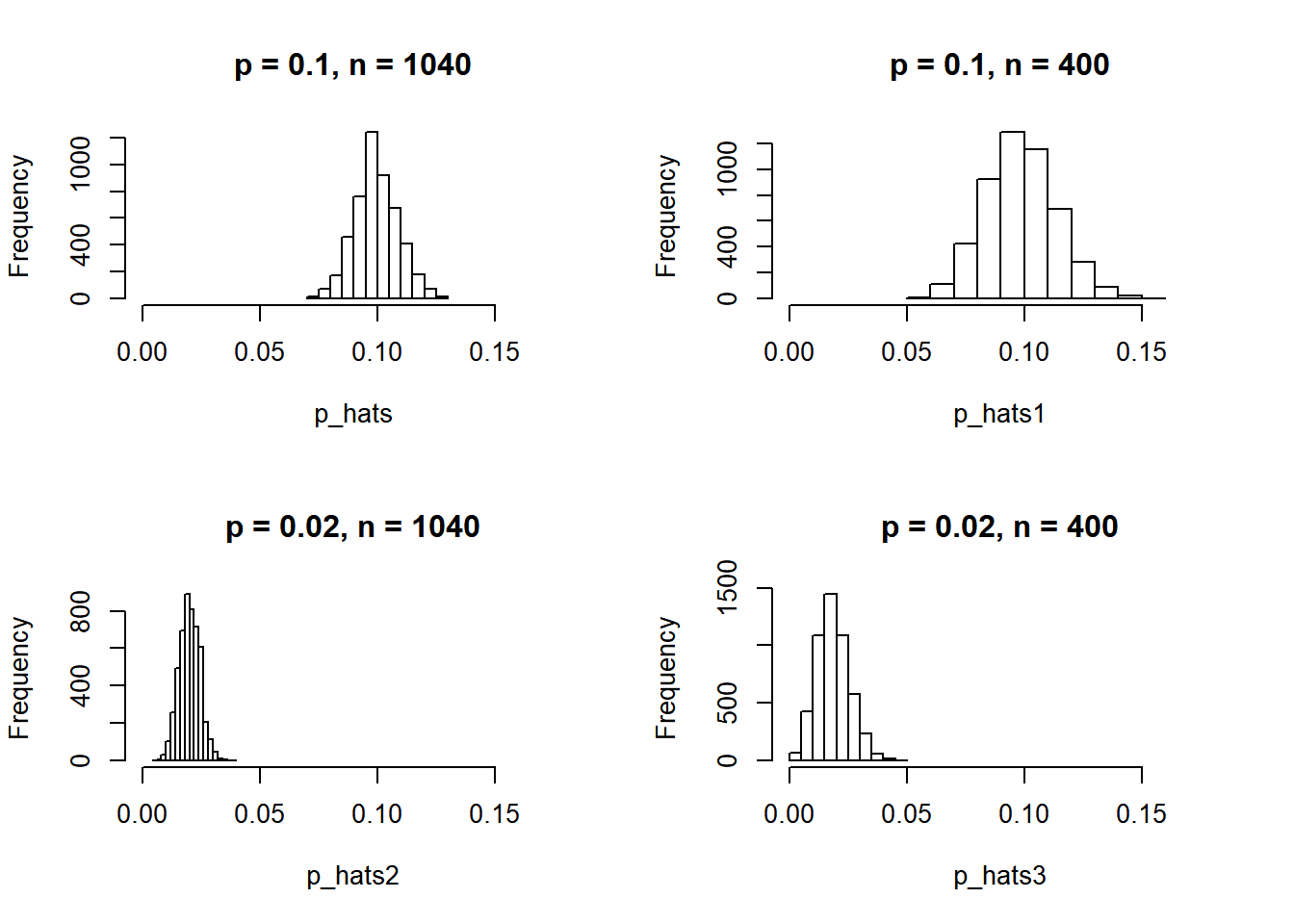par(mfrow = c(1, 1)) Once you’re done, you can reset the layout of the plotting window by using the command par(mfrow = c(1, 1)) command or clicking on “Clear All” above the plotting window (if using RStudio). Note that the latter will get rid of all your previous plots. 1. If you refer to Table 6, you’ll find that Australia has a sample proportion of 0.1 on a sample size of 1040, and that Ecuador has a sample proportion of 0.02 on 400 subjects. Let’s suppose for this exercise that these point estimates are actually the truth. Then given the shape of their respective sampling distributions, do you think it is sensible to proceed with inference and report margin of errors, as the reports does? # Australia n_au <- 1040 p_au <- 0.1 cond_au <- c(n_au * p_au >= 10, n_au * (1 - p_au) >= 10) # Ecuador n_ecu <- 400 p_ecu <- 0.02 cond_ecu <- c(n_ecu * p_ecu >= 10, n_ecu * (1 - p_ecu) >= 10) ans: n * p and TRUE n * ( 1 - p) => Based on Australia’s conditions => TRUE n * p and False n * ( 1 - p) => Based on Ecuador’s conditions => FALSE We can accept Australia and could reject Ecuador since it did not pass one of the conditions ## On your own The question of atheism was asked by WIN-Gallup International in a similar survey that was conducted in 2005. (We assume here that sample sizes have remained the same.) Table 4 on page 13 of the report summarizes survey results from 2005 and 2012 for 39 countries. • Answer the following two questions using the inference function. As always, write out the hypotheses for any tests you conduct and outline the status of the conditions for inference. ans: H0: There is no convincing evidence that Spain has change in its atheism index between 2005 and 2012 HA: There is convincing evidence that Spain has change in its atheism index between 2005 and 2012 **a.** Is there convincing evidence that Spain has seen a change in its atheism index between 2005 and 2012?\ *Hint:* Create a new data set for respondents from Spain. Form confidence intervals for the true proportion of athiests in both years, and determine whether they overlap. #country Spain for 2005 spain05 <- subset(atheism, nationality == "Spain" & year == "2005") head(spain05 , n=11) ## nationality response year ## 61188 Spain non-atheist 2005 ## 61189 Spain non-atheist 2005 ## 61190 Spain non-atheist 2005 ## 61191 Spain non-atheist 2005 ## 61192 Spain non-atheist 2005 ## 61193 Spain non-atheist 2005 ## 61194 Spain non-atheist 2005 ## 61195 Spain non-atheist 2005 ## 61196 Spain non-atheist 2005 ## 61197 Spain non-atheist 2005 ## 61198 Spain non-atheist 2005 spain05new <- atheism %>% filter(nationality == "Spain" & year == "2005") spain05new1<- table(spain05new ) propbraspain12_2005<- prop.table(spain05new1) propspain12_2005 <- data.frame(propbraspain12_2005) %>% filter (nationality == "Spain") #country Spain for 2012 spain12 <- subset(atheism, nationality == "Spain" & year == "2012") head(spain12 , n=11) ## nationality response year ## 45230 Spain non-atheist 2012 ## 45231 Spain non-atheist 2012 ## 45232 Spain non-atheist 2012 ## 45233 Spain non-atheist 2012 ## 45234 Spain non-atheist 2012 ## 45235 Spain non-atheist 2012 ## 45236 Spain non-atheist 2012 ## 45237 Spain non-atheist 2012 ## 45238 Spain non-atheist 2012 ## 45239 Spain non-atheist 2012 ## 45240 Spain non-atheist 2012 spain12new <- atheism %>% filter(nationality == "Spain" & year == "2012") spain12new1<- table(spain12new) propbraspain12_2012<- prop.table(spain12new1) propspain12_2012 <- data.frame(propbraspain12_2012) %>% filter (nationality == "Spain") propspain12_2005 ## nationality response year Freq ## 1 Spain atheist 2005 0.100349 ## 2 Spain non-atheist 2005 0.899651 propspain12_2012 ## nationality response year Freq ## 1 Spain atheist 2012 0.08995633 ## 2 Spain non-atheist 2012 0.91004367 #2 - sided inferences for Spain in year 2005 & 2012 spain <- subset(atheism, nationality == "Spain" & year == "2005" | nationality == "Spain" & year == "2012") inference(y = spain$response, x = spain$year, est = "proportion",type = "ht", null = 0, alternative = "twosided", method = "theoretical", success = "atheist") ## Warning: Explanatory variable was numerical, it has been converted to ## categorical. In order to avoid this warning, first convert your explanatory ## variable to a categorical variable using the as.factor() function. ## Response variable: categorical, Explanatory variable: categorical ## Two categorical variables ## Difference between two proportions -- success: atheist ## Summary statistics: ## x ## y 2005 2012 Sum ## atheist 115 103 218 ## non-atheist 1031 1042 2073 ## Sum 1146 1145 2291 ## Observed difference between proportions (2005-2012) = 0.0104 ## ## H0: p_2005 - p_2012 = 0 ## HA: p_2005 - p_2012 != 0 ## Pooled proportion = 0.0952 ## Check conditions: ## 2005 : number of expected successes = 109 ; number of expected failures = 1037 ## 2012 : number of expected successes = 109 ; number of expected failures = 1036 ## Standard error = 0.012 ## Test statistic: Z = 0.848 ## p-value = 0.3966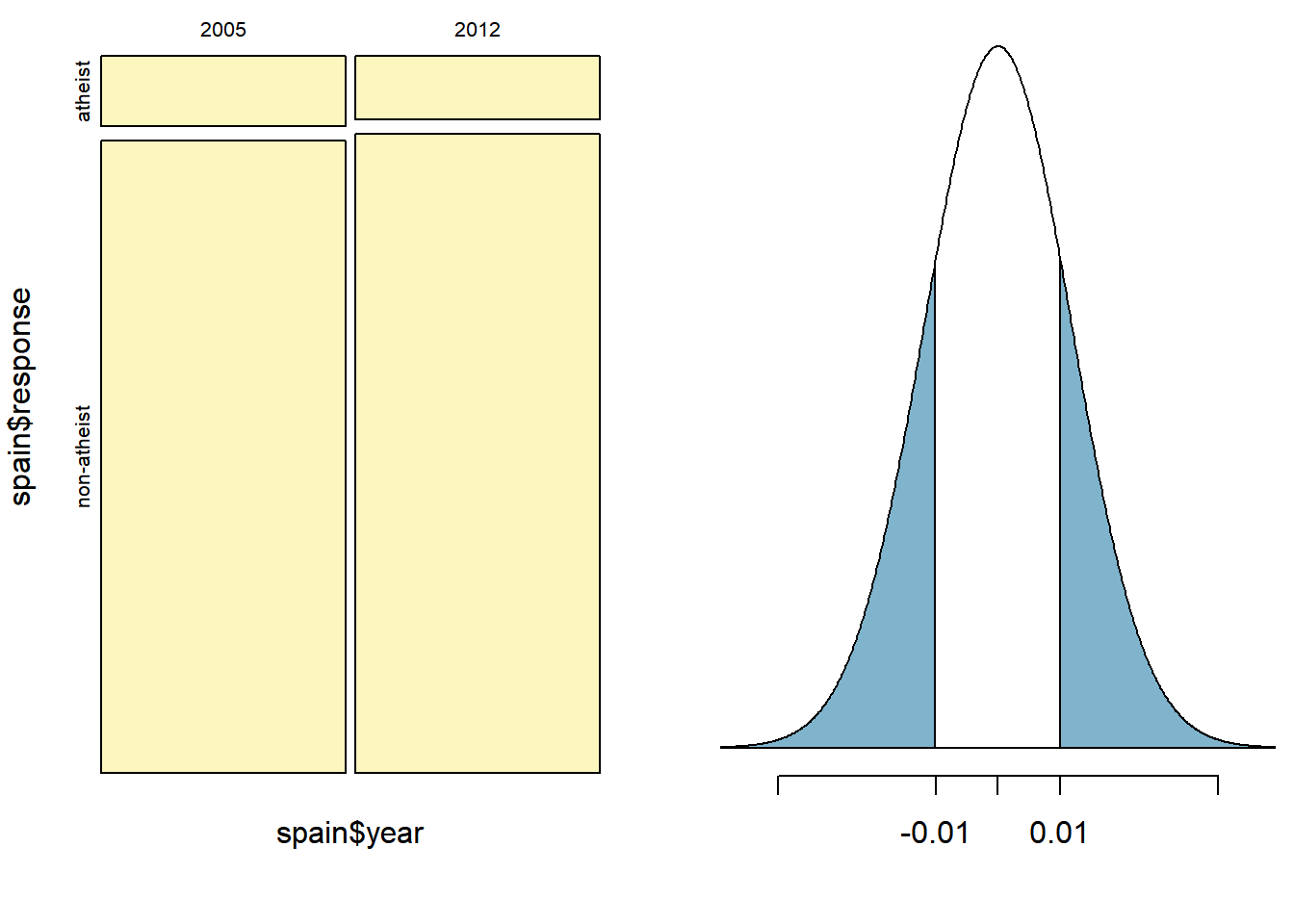ans: p-value = 0.3966 > 0.05, means H0 failed to be rejected. Therefore, no convincing evidence that Spain has change in its atheism index between 2005 and 2012 **b.** Is there convincing evidence that the United States has seen a change in its atheism index between 2005 and 2012? ans: We repeat the same process again but this time for the US: H0: There is no convincing evidence that USA has change in its atheism index between 2005 and 2012 HA: There is convincing evidence that USA has change in its atheism index between 2005 and 2012 #country US for 2005 us05 <- subset(atheism, nationality == "United States" & year == "2005") head(us05 , n=11) ## nationality response year ## 67899 United States non-atheist 2005 ## 67900 United States non-atheist 2005 ## 67901 United States non-atheist 2005 ## 67902 United States non-atheist 2005 ## 67903 United States non-atheist 2005 ## 67904 United States non-atheist 2005 ## 67905 United States non-atheist 2005 ## 67906 United States non-atheist 2005 ## 67907 United States non-atheist 2005 ## 67908 United States non-atheist 2005 ## 67909 United States non-atheist 2005 us05new <- atheism %>% filter(nationality == "United States" & year == "2005") us05new1 <- table(us05new ) propus05new1_2005<- prop.table(us05new1 ) propus05new1_2005 <- data.frame(propus05new1_2005) %>% filter (nationality == "United States") #country US for 2012 us12 <- subset(atheism, nationality == "United States" & year == "2012") head(us12 , n=11) ## nationality response year ## 49926 United States non-atheist 2012 ## 49927 United States non-atheist 2012 ## 49928 United States non-atheist 2012 ## 49929 United States non-atheist 2012 ## 49930 United States non-atheist 2012 ## 49931 United States non-atheist 2012 ## 49932 United States non-atheist 2012 ## 49933 United States non-atheist 2012 ## 49934 United States non-atheist 2012 ## 49935 United States non-atheist 2012 ## 49936 United States non-atheist 2012 usnew <- atheism %>% filter(nationality == "United States" & year == "2012") usnew1 <- table(usnew) propbrusnew1_2012<- prop.table(usnew1 ) propbrusnew1_2012<- data.frame(propbrusnew1_2012) %>% filter (nationality == "United States") propus05new1_2005 ## nationality response year Freq ## 1 United States atheist 2005 0.00998004 ## 2 United States non-atheist 2005 0.99001996 propbrusnew1_2012 ## nationality response year Freq ## 1 United States atheist 2012 0.0499002 ## 2 United States non-atheist 2012 0.9500998 #2 - sided inferences for USA in year 2005 & 2012 us <- subset(atheism, nationality == "United States" & year == "2005" | nationality == "United States" & year == "2012") inference(y = us$response, x = us\$year, est = "proportion",type = "ht", null = 0, alternative = "twosided", method = "theoretical", success = "atheist")
## Warning: Explanatory variable was numerical, it has been converted to
## categorical. In order to avoid this warning, first convert your explanatory
## variable to a categorical variable using the as.factor() function.
## Response variable: categorical, Explanatory variable: categorical
## Two categorical variables
## Difference between two proportions -- success: atheist
## Summary statistics:
##              x
## y             2005 2012  Sum
##   atheist       10   50   60
##   non-atheist  992  952 1944
##   Sum         1002 1002 2004
## Observed difference between proportions (2005-2012) = -0.0399
##
## H0: p_2005 - p_2012 = 0
## HA: p_2005 - p_2012 != 0
## Pooled proportion = 0.0299
## Check conditions:
##    2005 : number of expected successes = 30 ; number of expected failures = 972
##    2012 : number of expected successes = 30 ; number of expected failures = 972
## Standard error = 0.008
## Test statistic: Z =  -5.243
## p-value =  0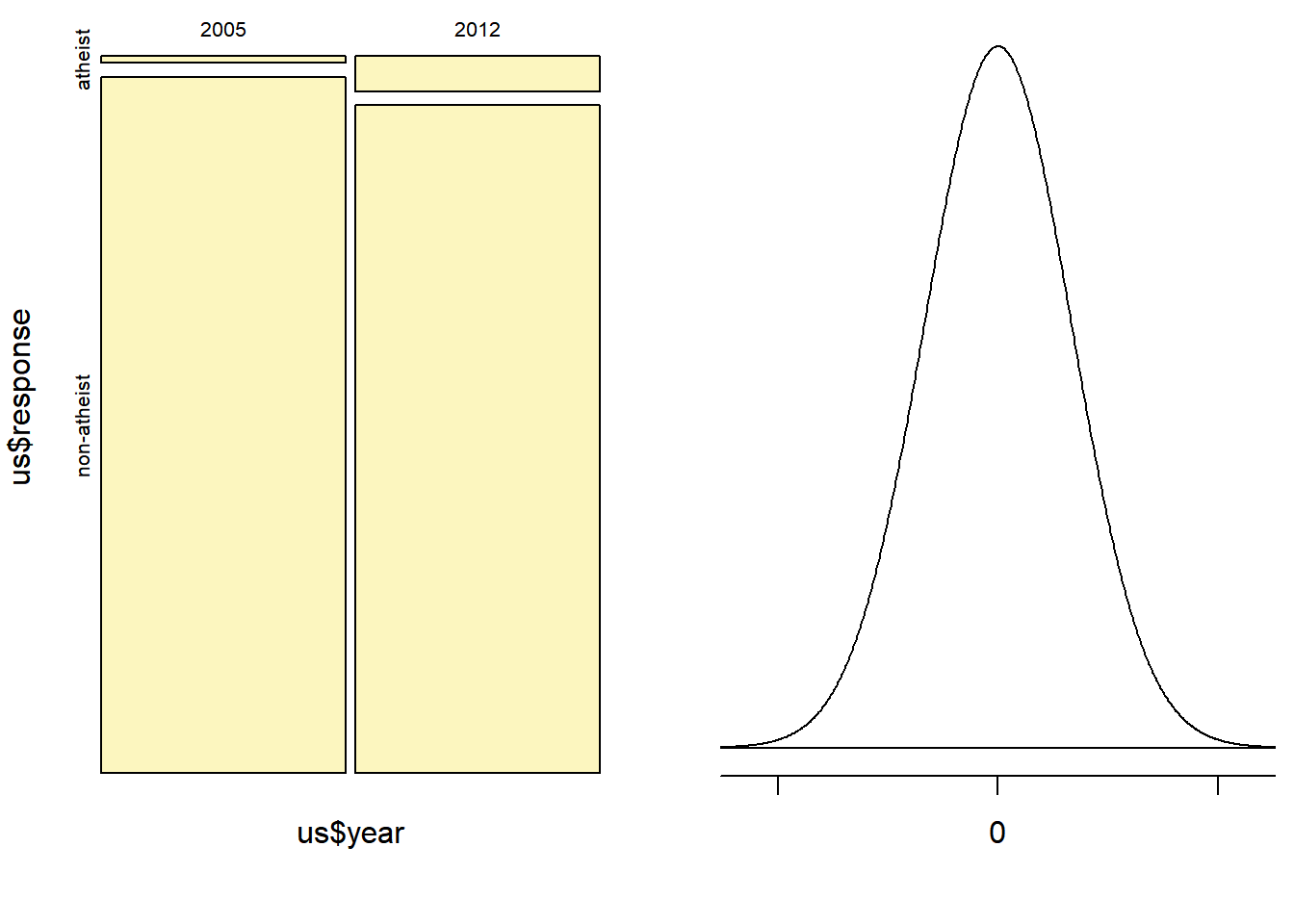ans: Since p-value = 0, we can reject the Ho and state that there is a change in atheism in the USA since 2005

• If in fact there has been no change in the atheism index in the countries listed in Table 4, in how many of those countries would you expect to detect a change (at a significance level of 0.05) simply by chance?
Hint: Look in the textbook index under Type 1 error.

ans:

A type 1 error is rejecting the null hypothesis when H0 is actually true.

Typically we do not want to incorrectly reject H0 more than 5% of the time; this resumes to a 0.05 significance

level. There are 39 countries listed in Table 4; all we multiply 0.05 by 39 to estimate

how many countries we would expect to detect a change in the atheism index simply by chance.

The result is about 2 countries would be expected to detect a change in atheism just by chance.

• Suppose you’re hired by the local government to estimate the proportion of residents that attend a religious service on a weekly basis. According to the guidelines, the estimate must have a margin of error no greater than 1% with 95% confidence. You have no idea what to expect for $$p$$. How many people would you have to sample to ensure that you are within the guidelines?
Hint: Refer to your plot of the relationship between $$p$$ and margin of error. Do not use the data set to answer this question.
p <-0.5 # Will generate the maximum ME
z <-1.96 # 95% Confidence interval
ME <- 0.01 # Marging of error no greater than 0.01
n <- p * (1 - p) * z ^ 2  / ME ^ 2
n
##  9604

ans:

Using the SE formula, we back tracked to calculate the estimated sample size of n. Since we know z=1.96, Margin

of error needed = 1% and from the plot we have p of 0.5 that gives the largest Margin of error. Therefore, based

on the calculation above, the sample size should be no less than 9604